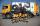Metal alloy

What is the ratio of metals in the alloy that is in the 50 tonnes of steel to 30 kg nickel?

Result

x =  0.06 %
y =  99.94 %
p =  0.0006004

Solution:

x = 100-y
x = 30/(50*1000)*100
p = 30/(50*1000-30)

x+y = 100
x = 0.06
p = 0.0006

p = 34997 ≈ 0.0006
x = 350 = 0.06
y = 499750 = 99.94

Calculated by our linear equations calculator.Leave us a comment of example and its solution (i.e. if it is still somewhat unclear...):Be the first to comment!To solve this example are needed these knowledge from mathematics:

Our percentage calculator will help you quickly calculate various typical tasks with percentages. Do you have a linear equation or system of equations and looking for its solution? Or do you have quadratic equation?

Next similar examples:

1. Family parcelsIn father will he divided the land so that the older son had three bigger part than younger son. Later elder son gave 2.5 ha field to younger and they had both the same. Determine the area of family parcel.
2. ClassroomThere are eighty more girls in the class than boys. Boys are 40 percent and girls are 60 percent. How many are boys and how many girls?
3. Barrel 4Barrel of water weighs 63 kg. After off 75% water, the weight of the barrel with water is 21 kg. How many kg weigh empty barrel and how many kgs water in it?
4. TheatroTheatrical performance was attended by 480 spectators. Women were in the audience 40 more than men and children 60 less than half of adult spectators. How many men, women and children attended a theater performance?
5. PercentCalculate how many % is the number 26.25 less than the number 105.
6. Collecting dayAt collecting day at school pupils of three classes took total of 1063 kg of paper. Class B collected half more than A Class and class C 55 kg more than B. How many kg of paper collected each class?
7. Alcohol mixtureFrom 55% and 80% alcohol we have to produce 0.2 kg of 60% alcohol. How many of them do we use in solution?
8. GirlsThe children's competition was attended by 63 girls, which is 30% of all children's participants. How many children attended this competition?
9. EquationsSolve following system of equations: 6(x+7)+4(y-5)=12 2(x+y)-3(-2x+4y)=-44
10. 13 ticketsA short and long sightseeing tour is possible at the castle. Ticket for a short sightseeing circuit costs CZK 60, for a long touring circuit costs CZK 100. So far, 13 tickets have been sold for 1140 CZK. How much did you pay for tickets for a short tour?
11. Substitutionsolve equations by substitution: x+y= 11 y=5x-25
12. PercentsHow many percents is 900 greater than the number 750?
13. PupilsThere are 350 girls in the school, and the other 30% of the total number of pupils are boys. How many pupils does the school have?
14. MushroomsMushrooms lose 90% by weight drying. How many fresh mushrooms are needed for 5 kg of dried mushrooms?
15. SchoolsThree schools are attended by 678 pupils. To the first attend 21 students more and to the third 108 fewer students than to second school. How many students attend the schools?
16. SummerjobThree students participated in the summerjob. Altogether they earn 1780, -. Peter got a third less than John and Paul got 100,- more than Peter. How much got every one of them?Calculate how many percent will increase the length of an HTML document, if any ASCII character unnecessarily encoded as hexadecimal HTML entity composed of six characters (ampersand, grid #, x, two hex digits and the semicolon). Ie. space as: &#x20;